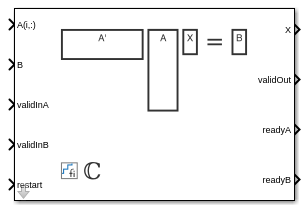Main Content

# Complex Partial-Systolic Matrix Solve Using Q-less QR Decomposition

Compute the value of X in A'AX = B for complex-valued matrices using Q-less QR decomposition

• Library:
• Fixed-Point Designer HDL Support / Matrices and Linear Algebra / Linear System Solvers

•## Description

The Complex Partial-Systolic Matrix Solve Using Q-less QR Decomposition block solves the system of linear equations, A'AX = B, using Q-less QR decomposition, where A and B are complex-valued matrices.

## Ports

### Input

expand all

Rows of matrix A, specified as a vector. A is an m-by-n matrix where m ≥ 2 and mn. If B is single or double, A must be the same data type as B. If A is a fixed point data type, A must be signed, use binary-point scaling, and have the same word length as B. Slope-bias representation is not supported for fixed-point data types.

Data Types: `single` | `double` | `fixed point`
Complex Number Support: Yes

Matrix B, specified as a vector. B is an m-by-p matrix where m ≥ 2. If A is single or double, B must be the same data type as A. If B is a fixed-point data type, B must be signed, use binary-point scaling, and have the same word length as A. Slope-bias representation is not supported for fixed-point data types.

Data Types: `single` | `double` | `fixed point`
Complex Number Support: Yes

Whether input A is valid, specified as a Boolean scalar. This control signal indicates when the data from the A(i,:) input port is valid. When this value is 1 (`true`) and the value at readyA is 1 (`true`), the block captures the values at the A(i,:) input port. When this value is 0 (`false`), the block ignores the input samples.

After sending a `true` validInA signal, there may be some delay before readyA is set to `false`. To ensure all data is processed, you must wait until readyA is set to `false` before sending another `true` validInA signal.

Data Types: `Boolean`

Whether input B is valid, specified as a Boolean scalar. This control signal indicates when the data from the B input port is valid. When this value is 1 (`true`) and the value at readyB is 1 (`true`), the block captures the values at the B input port. When this value is 0 (`false`), the block ignores the input samples.

After sending a `true` validInB signal, there may be some delay before readyB is set to `false`. To ensure all data is processed, you must wait until readyB is set to `false` before sending another `true` validInB signal.

Data Types: `Boolean`

Whether to clear internal states, specified as a Boolean scalar. When this value is 1 (`true`), the block stops the current calculation and clears all internal states. When this value is 0 (`false`) and the validIn value is 1 (`true`), the block begins a new subframe.

Data Types: `Boolean`

### Output

expand all

Matrix X, returned as a vector or matrix.

Data Types: `single` | `double` | `fixed point`

Whether the output data is valid, returned as a Boolean scalar. This control signal indicates when the data at the output port X is valid. When this value is 1 (`true`), the block has successfully computed a row of matrix X. When this value is 0 (`false`), the output data is not valid.

Data Types: `Boolean`

Whether the block is ready for input A, returned as a Boolean scalar. This control signal indicates when the block is ready for new input data. When this value is 1 (`true`) and validInA value is 1 (`true`), the block accepts input data in the next time step. When this value is 0 (`false`), the block ignores input data in the next time step.

After sending a `true` validInA signal, there may be some delay before readyA is set to `false`. To ensure all data is processed, you must wait until readyA is set to `false` before sending another `true` validInA signal.

Data Types: `Boolean`

Whether the block is ready for input B, returned as a Boolean scalar. This control signal indicates when the block is ready for new input data. When this value is 1 (`true`) and validInB value is 1 (`true`), the block accepts input data in the next time step. When this value is 0 (`false`), the block ignores input data in the next time step.

After sending a `true` validInB signal, there may be some delay before readyB is set to `false`. To ensure all data is processed, you must wait until readyB is set to `false` before sending another `true` validInB signal.

Data Types: `Boolean`

## Parameters

expand all

Number of rows in matrix A, specified as a positive integer-valued scalar.

#### Programmatic Use

 Block Parameter: `m` Type: character vector Values: positive integer-valued scalar Default: `4`

Number of columns in matrix A and rows in matrix B, specified as a positive integer-valued scalar.

#### Programmatic Use

 Block Parameter: `n` Type: character vector Values: positive integer-valued scalar Default: `4`

Number of columns in matrix B, specified as a positive integer-valued scalar.

#### Programmatic Use

 Block Parameter: `p` Type: character vector Values: positive integer-valued scalar Default: `1`

Data type of the output matrix X, specified as `fixdt(1,18,14)`, `double`, `single`, `fixdt(1,16,0)`, or as a user-specified data type expression. The type can be specified directly, or expressed as a data type object such as `Simulink.NumericType`.

#### Programmatic Use

 Block Parameter: `OutputType` Type: character vector Values: `'fixdt(1,18,14)'` | `'double'` | `'single'` | `'fixdt(1,16,0)'` | `''` Default: `'fixdt(1,18,14)'`

expand all

## Extended Capabilities

Introduced in R2020b

## SupportGet trial now